# Java中的HashMap

## 扩容机制

### jdk1.8

1. 统计每个下标位置的元索个数。
2. 如果该位下的元素个数超过了8，则生成一个新的红黑树，开将根节点添加到新数组的对应位置。
3. 如果该位置下的元素个数没有超过8，那么则生成一个链表，开将链表头节点添加数组的对应位置。

## jdk1.7中HashMap死循环问题

import java.util.HashMap;
import java.util.Map;

static Map<String, String> map = new HashMap<>();

int start = 0;

this.start = start;
}

@Override
public void run() {
for (int i = start; i < 100000; i += 2) {
map.put(Integer.toString(i), Integer.toBinaryString(i));
}
}
}

public static void main(String[] args) throws InterruptedException {
t1.start();
t2.start();
t1.join();
t2.join();
System.out.println(map.size());
}
}



    void transfer(Entry[] newTable, boolean rehash) {
int newCapacity = newTable.length;
for (Entry<K,V> e : table) {
while(null != e) {
Entry<K,V> next = e.next;
if (rehash) {
e.hash = null == e.key ? 0 : hash(e.key);
}
int i = indexFor(e.hash, newCapacity);
e.next = newTable[i];
newTable[i] = e;
e = next;
}
}
}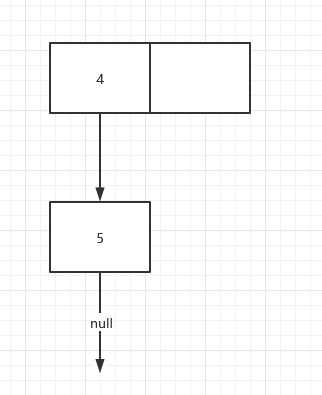Entry<K,V> next = e.next;


e = 4节点
next = 5节点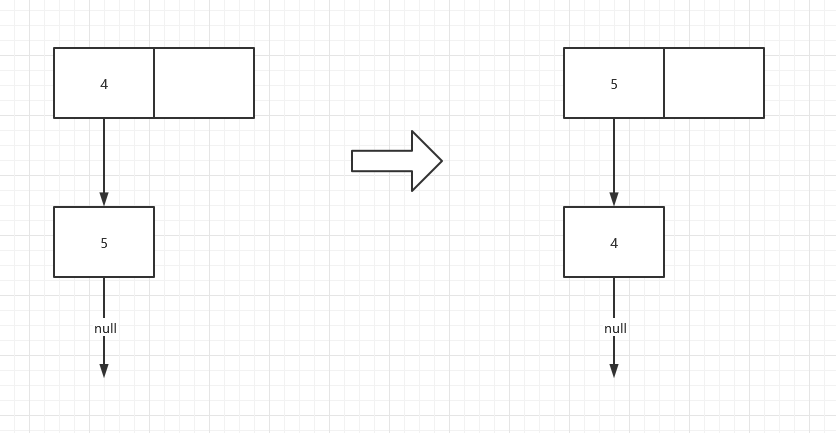Entry<K,V> next = e.next;


e = 4号节点,
next = 5号节点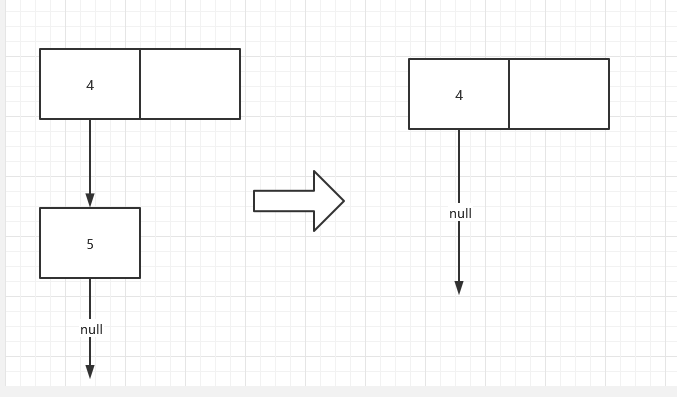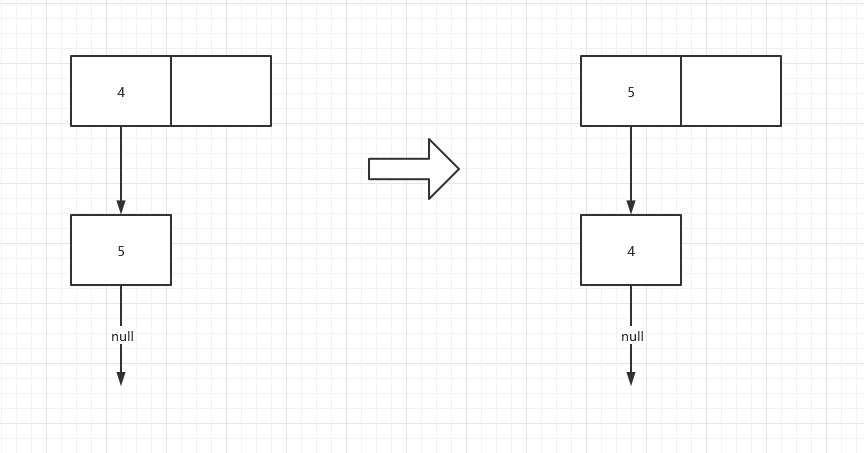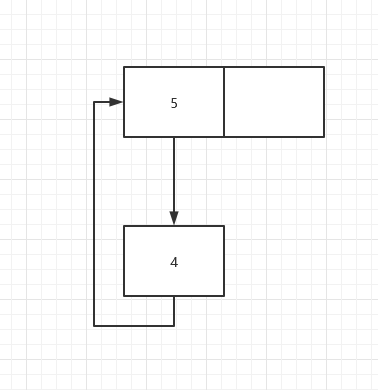## HashMap中的一些参数

### 初始容量

    static final int DEFAULT_INITIAL_CAPACITY = 1 << 4; // aka 16


### 最大容量

    static final int MAXIMUM_CAPACITY = 1 << 30;


### 扩容因子

static final float DEFAULT_LOAD_FACTOR = 0.75f;


static int indexFor(int h, int length) {
// assert Integer.bitCount(length) == 1 : "length must be a non-zero power of 2";
return h & (length-1);
}


hashMap用位运算来替代取模运算(capacity - 1) & hash这种运算方式为什么可以得到跟取模一样的结果呢？ 答案是capacity是2的N次幂，计算机做位运算的效率远高于做取模运算的效率，测试见：位运算和取模运算的运算效率对比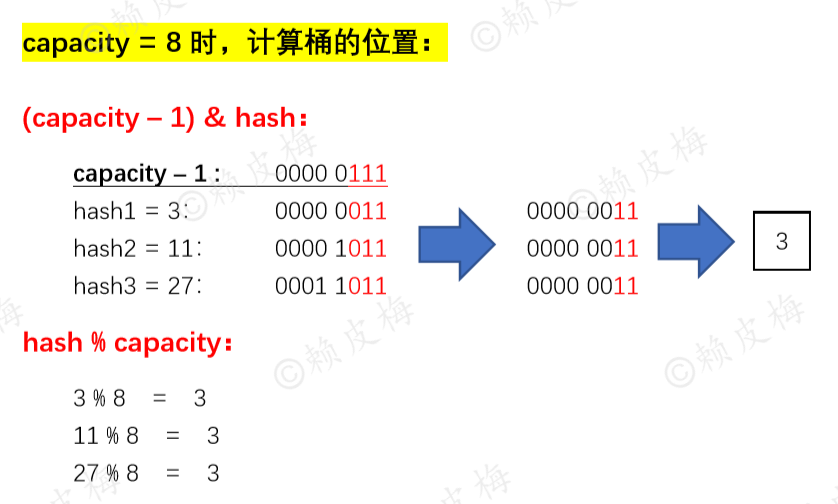final Node<K,V>[] resize() {
Node<K,V>[] oldTab = table;
int oldCap = (oldTab == null) ? 0 : oldTab.length;
int oldThr = threshold;
int newCap, newThr = 0;
if (oldCap > 0) {
if (oldCap >= MAXIMUM_CAPACITY) {
threshold = Integer.MAX_VALUE;
return oldTab;
}
else if ((newCap = oldCap << 1) < MAXIMUM_CAPACITY &&
oldCap >= DEFAULT_INITIAL_CAPACITY)
newThr = oldThr << 1; // double threshold
}
else if (oldThr > 0) // initial capacity was placed in threshold
newCap = oldThr;
else {               // zero initial threshold signifies using defaults
newCap = DEFAULT_INITIAL_CAPACITY;
}
if (newThr == 0) {
float ft = (float)newCap * loadFactor;
newThr = (newCap < MAXIMUM_CAPACITY && ft < (float)MAXIMUM_CAPACITY ?
(int)ft : Integer.MAX_VALUE);
}
threshold = newThr;
@SuppressWarnings({"rawtypes","unchecked"})
Node<K,V>[] newTab = (Node<K,V>[])new Node[newCap];
table = newTab;
if (oldTab != null) {
for (int j = 0; j < oldCap; ++j) {
Node<K,V> e;
if ((e = oldTab[j]) != null) {
oldTab[j] = null;
if (e.next == null)
newTab[e.hash & (newCap - 1)] = e;
else if (e instanceof TreeNode)
((TreeNode<K,V>)e).split(this, newTab, j, oldCap);
else { // preserve order
Node<K,V> loHead = null, loTail = null;
Node<K,V> hiHead = null, hiTail = null;
Node<K,V> next;
do {
next = e.next;
if ((e.hash & oldCap) == 0) {
if (loTail == null)
else
loTail.next = e;
loTail = e;
}
else {
if (hiTail == null)
else
hiTail.next = e;
hiTail = e;
}
} while ((e = next) != null);
if (loTail != null) {
loTail.next = null;
}
if (hiTail != null) {
hiTail.next = null;
}
}
}
}
}
return newTab;
}


## 参考资料

jdk1.8 HashMap底层数据结构：深入解析为什么jdk1.8 HashMap的容量一定要是2的n次幂

posted @ 2021-10-05 20:12  Grey Zeng  阅读(250)  评论(0编辑  收藏  举报